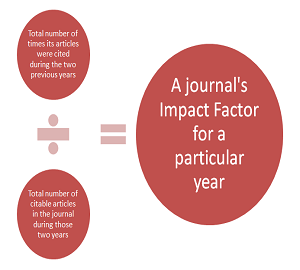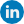The Impact Factor of scientific journals

Impact Factor indicates the average number of citations of the journal’s articles compared to other journals and scientific references.Journalimpactfactor

Therefore, this factor in fact illustrates the validity of that journal in its research area among other journals. (A journal with a higher impact factor is more important than a journal with a lower impact factor). Impact factor was defined by the founder of the ISI Institute. However, in 1992, the company was purchased by another company called Thomson ISI.

Determination of the impact factor

Impact factor means how much a journal influences the world of science and in fact reflects the validity of a journal.

How to calculate impact factor

The number of citations of articles during a two-year period is computed first. Then, the number of published articles of that journal during that two years is extracted, and the first number is divided by the second number. For example, if you want to determine the impact factor of a journal in 2014, extract the citations from 2013 and 2012 (for example, 30), and divide it by the number of articles published in these two years (for example, 10). The impact factor obtained is 3.Before you leave